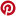# Trigonometric Ratios of Special Angles: 0, 30, 45, 60, 90

Videos, worksheets, solutions, and activities to help students learn how to find the Trigonometric Ratios of Special Angles: 0, 30, 45, 60, 90.

Related Topics:
More Grade 9 Math Lessons
More Trigonometry Lessons
Trigonometry Worksheets
Trigonometry Games

How to derive and memorize the trigonometric ratios of the special angles: 30, 45, 60?

The following special angles chart show how to derive the trig ratios of 30, 45 and 60 from the 30-60-90 and 45-45-90 special triangles. Scroll down the page if you need more examples and explanations on how to derive and use the trig ratios of special angles.Trigonometric Function Values of Special Angles
How to derive the trigonometric function values of 30, 45, and 60 degrees and their corresponding radian measure. Cofunction identities are also discussed:
sin θ = cos(90° - θ)
cos θ = sin(90° - θ)

How to find Trig Ratios of Special Angles? Part 1
This lesson shows how to find the trig ratios of the special angles: sine, cosine and tangent values of 0, 30, 45, 60 and 90 degrees. Scroll down the page for part 2. How to use the trig ratios of special angles to find exact values of expressions?
How to use the trig ratios of special angles to find exact values of expressions involving sine, cosine and tangent values of 0, 30, 45, 60 and 90 degrees?
Example:
Determine the exact values of each of the following:
a) sin30°tan45° + tan30°sin60°
b) cos30°sin45° + sin30°tan30° How to remember the Trig Ratios for Special Angles?
A Finger Trick for Trigonometry
If we insist that students memorize the values of sine and cosine for the basic angles 0, 30, 45, 60 and 90 degrees, then here's a cute little trick for doing so using the fingers on your hand. Cool Pattern for Trig Special Angles
A pattern to help you remember the Sine and Cosines of Special Angles in the first quadrant. 0, 30, 45, 60, 90 degrees.

How to evaluate trig functions of special angles?
Easy way to use right triangle and label sides to find sin, cos, tan, cot, csc, and sec of the special angles, and of angles at multiples of 90°. This is Part 1. Scroll down the page for part 2.
Example:
Find cos 90, tan 90, sin 630, sin 135, tan (-405), sin 210, tan (-30). Trigonometric functions of special angles, part 2
Example:
Find cos 300, cot 180, sin 1305, sec(-210), csc 750, cos 270, sin(-420).

Rotate to landscape screen format on a mobile phone or small tablet to use the Mathway widget, a free math problem solver that answers your questions with step-by-step explanations.

You can use the free Mathway calculator and problem solver below to practice Algebra or other math topics. Try the given examples, or type in your own problem and check your answer with the step-by-step explanations.